# GRE Subject Test: Math : Determinants

## Example Questions

### Example Question #1 : Determinants

Given the following matrix, find the determinant, if possible.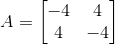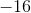There is no determinant.Explanation:

Write the formula to find the determinant given a 2 by 2 matrix.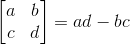Substituting in the given matrix we are able to find the determinant.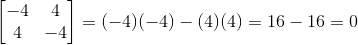### Example Question #191 : Algebra

Evaluate the determinant of the following matrix.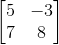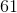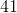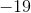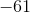Explanation:

Remember, to evaluate the determinant of a matrix use the following: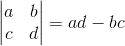The first step would be to write the determinant of the matrix: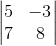Now we can evaluate: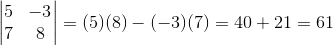### Example Question #1 : Determinants

Evaluate the determinant of the following matrix.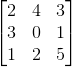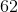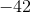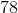Explanation:

To find the determinant of a 3 x 3 matrix, we must use the following: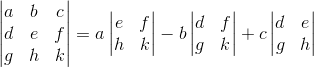The first thing we must do is write the determinant: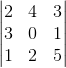Now we can proceed to evaluate the determinant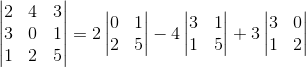Notice that the numbers 2, 4, and 3 are being multiplied by the determinants of the 2x2 matrices so we have: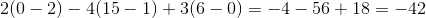### Example Question #191 : Algebra

Find the determinant of the matrix: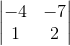Explanation:

Step 1: We need to recall how to find the determinant of a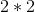matrix. To find the determinant of amatrix, we need to use the equation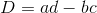, where=Determinant, and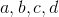are from the matrix.

Step 2: Identify a,b,c, and d in the original matrix.
a=first number on top row, b=second number on top row (next to a), c=first number on the bottom row, and d is the second number on the bottom row (next to c).

In this matrix, a=, b=, c=, and d=.

Step 3: Substitute the values of a,b,c, and d into the equation to find the determinant of the matrix.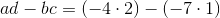We will simplify the right side.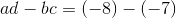. We see that there are two negative signs in the middle, which will become a plus sign.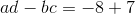. Simplify the right side.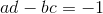is the determinant.

The determinant of the matrix is.

### Example Question #2 : Determinants

Find the determinate of Matrix A.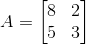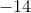Explanation:

Matrix A is given below.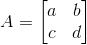The formula for the determinate of a 2x2 matrix is: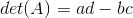Plugging in the values gives us: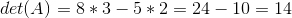### All GRE Subject Test: Math Resources